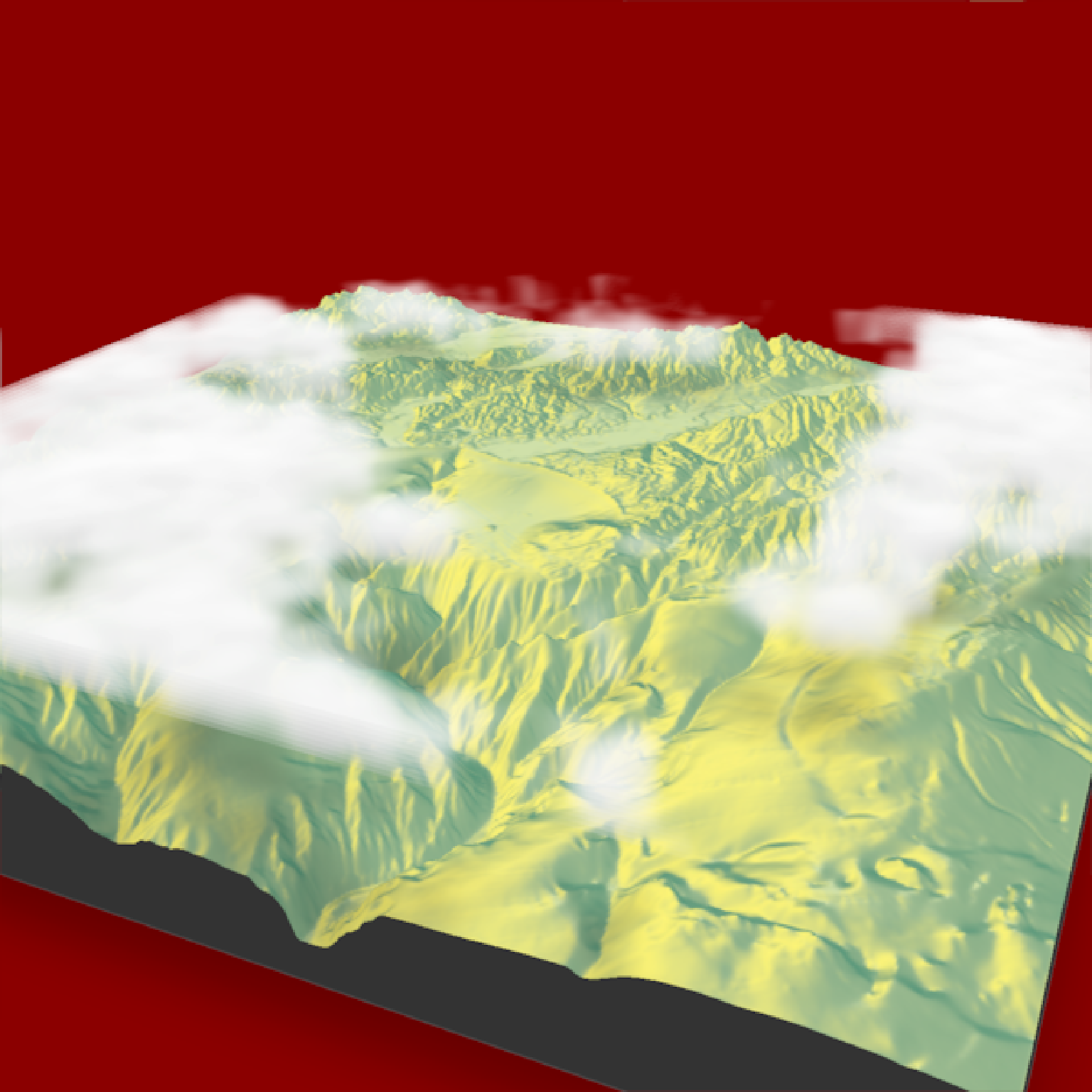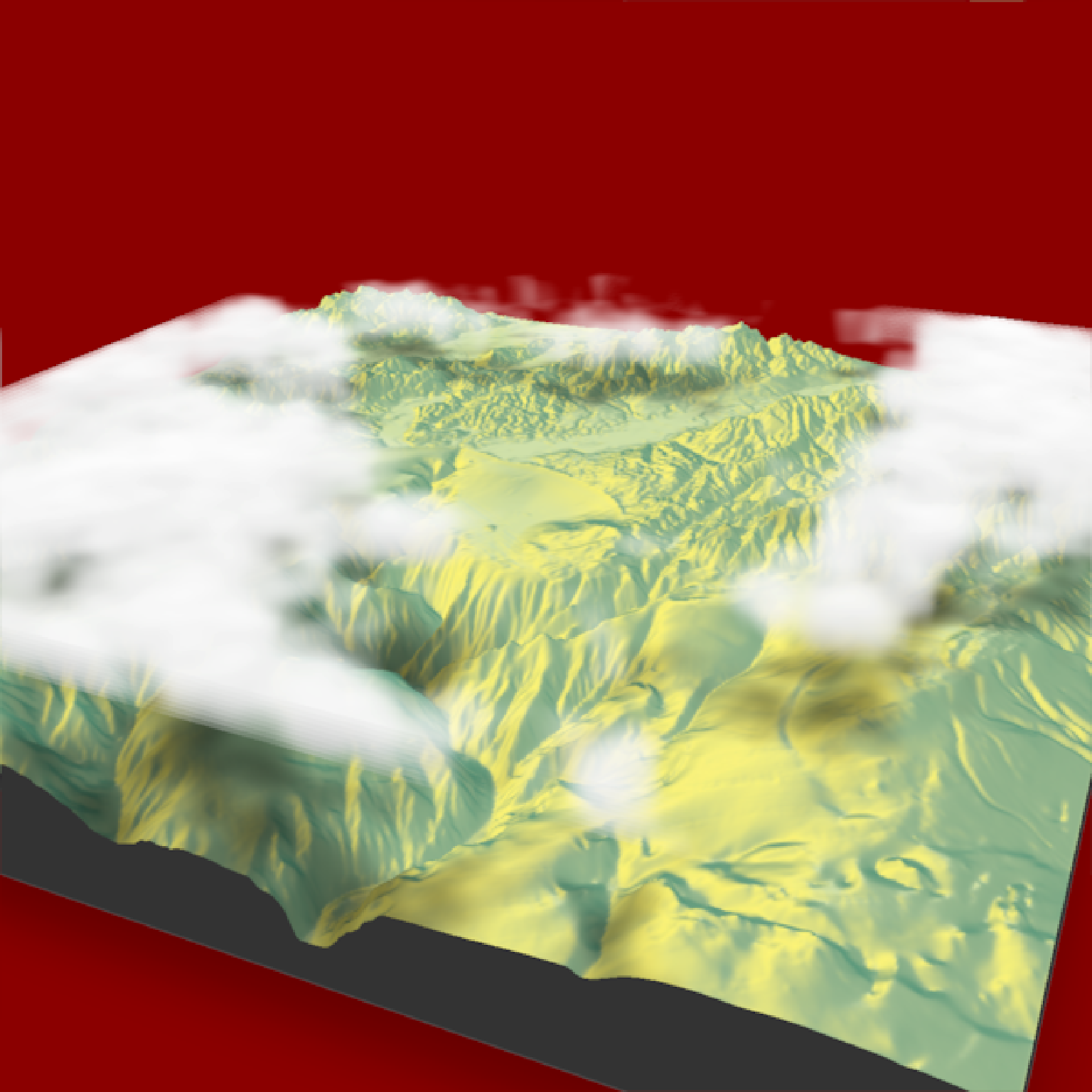Render shadows from the 3D floating cloud layer on the ground. Use this function to add shadows to the map with the add_shadow() function.

For realistic results, argument should match those passed to render_clouds(). The exception to this is attenuation_coef, which can be used to adjust the darkness of the resulting shadows.

cloud_shade(
heightmap,
start_altitude = 1000,
end_altitude = 2000,
sun_altitude = 90,
sun_angle = 315,
time = 0,
cloud_cover = 0.5,
layers = 10,
offset_x = 0,
offset_y = 0,
scale_x = 1,
scale_y = 1,
scale_z = 1,
frequency = 0.005,
fractal_levels = 16,
attenuation_coef = 1,
seed = 1,
zscale = 1
)

## Arguments

heightmap

A two-dimensional matrix, where each entry in the matrix is the elevation at that point. This is used by render_clouds() to calculate the regions the clouds should be rendered in.

start_altitude

Default 1000. The bottom of the cloud layer.

end_altitude

Default 2000. The top of the cloud layer.

sun_altitude

Default 10. The angle, in degrees (as measured from the horizon) from which the light originates.

sun_angle

Default 315 (NW). The angle, in degrees, around the matrix from which the light originates. Zero degrees is North, increasing clockwise

time

Default 0. Advance this to make the clouds evolve and change in shape.

cloud_cover

Default 0.5. The percentage of cloud cover.

layers

Default 90. The number of layers to render the cloud layer.

offset_x

Default 0. Change this to move the cloud layer sideways.

offset_y

Default 0. Change this to move the cloud layer backwards and forward

scale_x

Default 1. Scale the fractal pattern in the x direction.

scale_y

Default 1. Scale the fractal pattern in the y direction.

scale_z

Default 1. Scale the fractal pattern in the z (altitude) direction. (automatically calculated). Scale the fractal pattern in the z (vertical) direction. s.

frequency

Default 0.005. The base frequency of the noise used to calculate the fractal cloud structure.

fractal_levels

Default 16. The fractal dimension used to calculate the noise. Higher values give more fine structure, but take longer to calculate.

attenuation_coef

Default 1. Amount of attenuation in the cloud (higher numbers give darker shadows). This value is automatically scaled to account for increasing the number of layers.

seed

Default 1. Random seed used to generate clouds.

zscale

Default 1. The ratio between the x and y spacing (which are assumed to be equal) and the z axis. For example, if the elevation levels are in units of 1 meter and the grid values are separated by 10 meters, zscale would be 10.

## Examples

if(run_documentation()) {
#Render clouds with cloud shadows on the ground
montereybay  %>%
plot_3d(montereybay,background="darkred",zscale=50)
render_camera(theta=-65, phi = 25, zoom = 0.45, fov = 80)
render_clouds(montereybay, zscale=50)
render_snapshot()
}if(run_documentation()) {
#Adjust the light direction for shadows and increase the attenuation for darker clouds
montereybay  %>%